# Brake

Brake based on Coulomb friction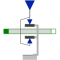# Information

This information is part of the Modelica Standard Library maintained by the Modelica Association.

This component models a brake, i.e., a component where a frictional force is acting between the housing and a flange and a controlled normal force presses the flange to the housing in order to increase friction. The normal force fn has to be provided as input signal f_normalized in a normalized form (0 ≤ f_normalized ≤ 1), fn = fn_max*f_normalized, where fn_max has to be provided as parameter. Friction in the brake is modelled in the following way:

When the absolute velocity "v" is not zero, the friction force is a function of the velocity dependent friction coefficient mue(v) , of the normal force "fn", and of a geometry constant "cgeo" which takes into account the geometry of the device and the assumptions on the friction distributions:

```        frictional_force = cgeo * mue(v) * fn
```

Typical values of coefficients of friction:

```      dry operation   :  mue = 0.2 .. 0.4
operating in oil:  mue = 0.05 .. 0.1
```

The positive part of the friction characteristic mue(v), v >= 0, is defined via table mue_pos (first column = v, second column = mue). Currently, only linear interpolation in the table is supported.

When the absolute velocity becomes zero, the elements connected by the friction element become stuck, i.e., the absolute position remains constant. In this phase the friction force is calculated from a force balance due to the requirement, that the absolute acceleration shall be zero. The elements begin to slide when the friction force exceeds a threshold value, called the maximum static friction force, computed via:

```       frictional_force = peak * cgeo * mue(w=0) * fn   (peak >= 1)
```

This procedure is implemented in a "clean" way by state events and leads to continuous/discrete systems of equations if friction elements are dynamically coupled. The method is described in:

Otter M., Elmqvist H., and Mattsson S.E. (1999):
Hybrid Modeling in Modelica based on the Synchronous Data Flow Principle. CACSD'99, Aug. 22.-26, Hawaii.

More precise friction models take into account the elasticity of the material when the two elements are "stuck", as well as other effects, like hysteresis. This has the advantage that the friction element can be completely described by a differential equation without events. The drawback is that the system becomes stiff (about 10-20 times slower simulation) and that more material constants have to be supplied which requires more sophisticated identification. For more details, see the following references, especially (Armstrong and Canudas de Wit 1996):

Armstrong B. (1991):
Control of Machines with Friction. Kluwer Academic Press, Boston MA.

Armstrong B., and Canudas de Wit C. (1996):
Friction Modeling and Compensation. The Control Handbook, edited by W.S.Levine, CRC Press, pp. 1369-1382.

Canudas de Wit C., Olsson H., Åström K.J., and Lischinsky P. (1995):
A new model for control of systems with friction. IEEE Transactions on Automatic Control, Vol. 40, No. 3, pp. 419-425.

# Parameters (7)

useSupport Value: false Type: Boolean Description: = true, if support flange enabled, otherwise implicitly grounded Value: false Type: Boolean Description: =true, if heatPort is enabled Value: 1e-3 Type: Velocity (m/s) Description: Relative velocity near to zero (see model info text) Value: [0, 0.5] Type: Real[:,2] Description: [v, f] Positive sliding friction characteristic (v>=0) Value: 1 Type: Real Description: Peak for maximum value of mue at w==0 (mue0_max = peak*mue_pos[1,2]) Value: 1 Type: Real Description: Geometry constant containing friction distribution assumption Value: Type: Force (N) Description: Maximum normal force

# Connectors (5)

flange_a flange_b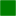Type: Flange_a Description: Flange of left shaft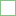Type: Flange_b Description: Flange of right shaft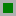Type: Support Description: Support/housing of component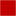Type: HeatPort_a Description: Optional port to which dissipated losses are transported in form of heat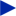Type: RealInput Description: Normalized force signal 0..1 (normal force = fn_max*f_normalized; brake is active if > 0)

# Used in Examples (2)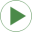Brake Modelica.Mechanics.Translational.Examples Demonstrate braking of a translational moving massHeatLosses Modelica.Mechanics.Translational.Examples Demonstrate the modeling of heat losses